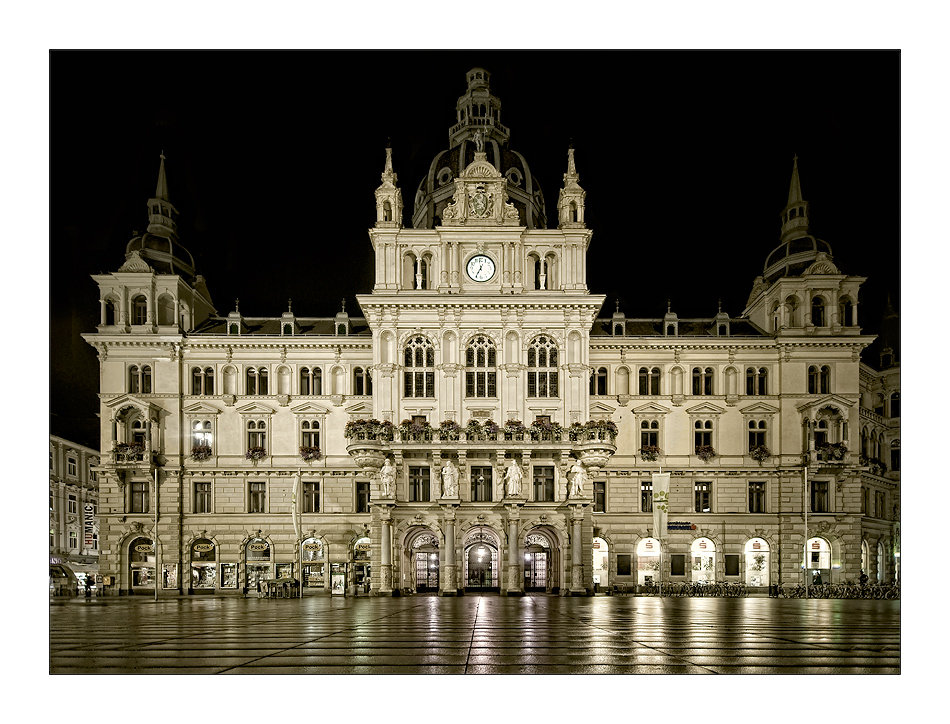# Infinite Balanced CoverOur Mission:

to the Forefront of International Research
and to stabilize this statusInfinite Balanced Cover Introduction. To emphasize the following problem's significance, we point out that, for the 2020 complex quadratic fields K = Q(D1/2) with discriminant -106 < D < 0 and 3-class group Cl3(K) of type (3,3), our extensive computations of 2010 [Ma1] and our theories developed in 2009 - 2013 [Ma4] have revealed that the dominant part of exactly 936 fields has a 3-class tower of length l3(K) = 2 (minimum |D| = 4027), the second largest part of at least 411 fields has a 3-class tower of length l3(K) = 3 [BuMa] (minimum |D| = 9748), the third largest part of 297 fields is of the kind described by Theorem H.4-GS, which characterizes the ground state of capitulation type H.4 [Ma2] (minimum |D| = 3896). Theorem H.4-GS. (D. C. Mayer, 2009 [Ma3]) For a complex quadratic field K = Q(D1/2), D < 0, with 3-class group Cl3(K) of type (3,3) the following conditions are equivalent: The 3-class groups of the four unramified cyclic cubic extensions N1,…,N4 of K are of the types Cl3(N1) ≅ (3,9) and Cl3(Ni) ≅ (3,3,3) for 2 ≤ i ≤ 4 (up to the selection of an ordering). The Galois group of the second Hilbert 3-class field F32(K), that is the maximal metabelian unramified 3-extension of K, is isomorphic to the unbalanced 3-group SmallGroup(729,45) of class 4, coclass 2, and derived length 2. Problem. (originally intended for the problem sessions of WCNT 2013 [Ma5]) By which arithmetical criteria can we decide for a complex quadratic field of the kind described by Theorem H.4-GS which of the infinitely many non-metabelian (balanced) Schur σ-groups Gn, n ≥ 2, given by L. Bartholdi and M. R. Bush [BtBu] (2007), is isomorphic to the Galois group of the maximal unramified pro-3 extension F3∞(K) of K ? Remark. The group Gn, n ≥ 2, has second derived quotient Gn / Gn'' ≅ SmallGroup(729,45) [we express this fact by saying that the set { Gn | n ≥ 2 } is the infinite balanced cover of SmallGroup(729,45)] and is of order 33n+2, class 2n + 1, coclass n + 1, and of unbounded derived length floor(log2(3n+3)) for n → ∞.